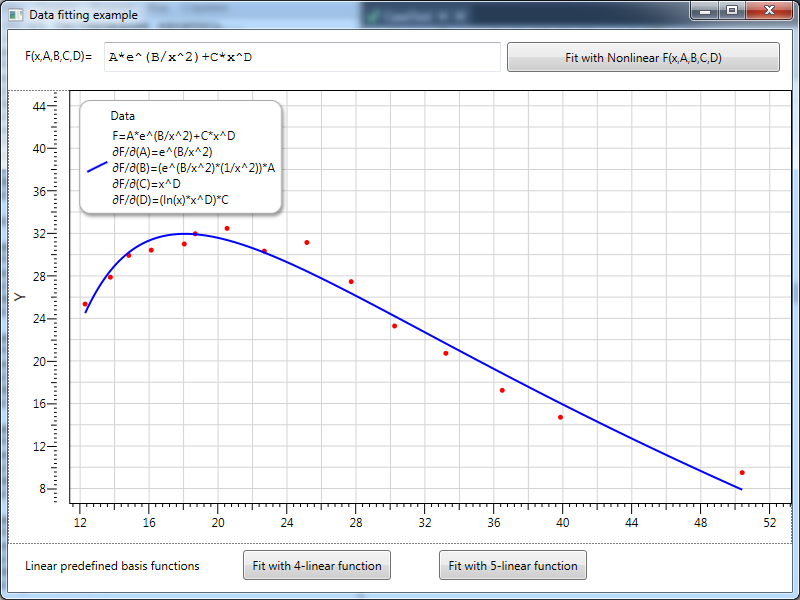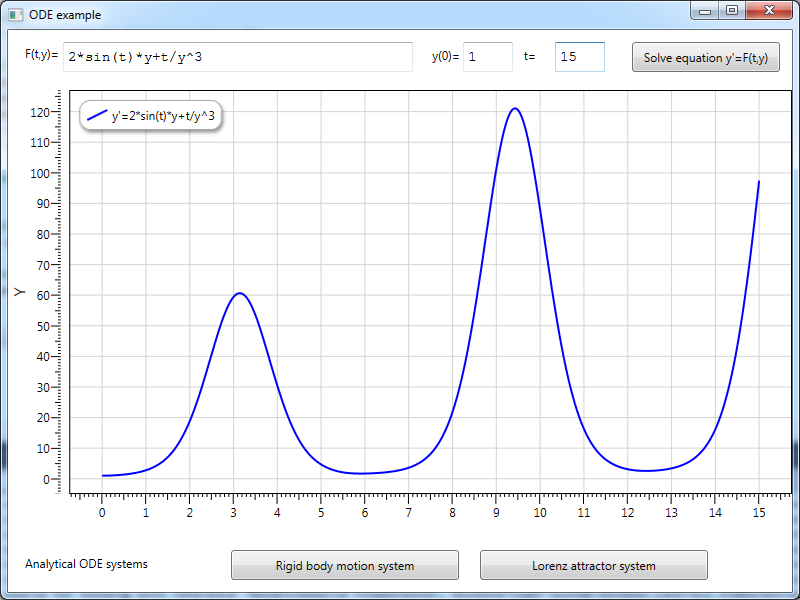Extreme ANALYTICS – is a plugin for computational library Extreme Optimization (.NET Math and Statistics Library from http://www.extremeoptimization.com) that introduces analytical capabilities to it.

The advantages of using analytical capabilities are:

- Writing code without special classes for calculation delegates;

- Easily storing and transmitting data as strings;

- Convenient data presentation for developer and user;

- Automatically calculated derivatives for numerical algorithms.

- Parameterized problems solution.

Extreme ANALYTICS provides integration between Extreme and ANALYTICS libraries. The following extensions realized: Evaluation, Calculus, ODE and Optimization. Evaluation assembly realizes total analytical calculation engine for Extreme double and complex vectors and matrices. Calculus assembly contains special classes of Analytical functions those provide evaluation methods from analytical expressions, including Analytical Derivatives calculation. Optimization assembly contains Analytical descendants of linear basis and nonlinear curve for fitting data with arbitrary functions set up in Analytical form. ODE assembly realizes special classes for ODE system set up in Analytical form.

Extreme ANALYTICS Source code example (VS 2010 solution): ExtremeAnalytics.Demo.rar

ExtremeAnalytics Evaluation

Evaluation assembly contains realization of special Translator class. This class registers analytical operations with Extreme double and complex vectors and matrices.  Registered operations include:
- standard algebraic operators (+, -, *, /, ^);
- special vector operators (dot product);
- transcendental functions (sin, cos, exp, log and so on);
- basic special functions (abs, floor and so on).

ExtremeAnalytics Calculus

Calculus assembly provides possibilities for differentiation and integration of functions set up in analytical form. These functions can be provided by user via simple string input. Realized Analytical function classes allows calculate Analytical derivative expressions that can be used then for evaluation purposes. For integration algorithms, the classes provide evaluation of analytical expression in any integration node.

ExtremeAnalytics Optimization

Optimization assembly introduces Analytical descendant classes of linear basis and nonlinear curve. Analytical classes allows construction of bases and curves from string expressions (formulae) and then use evaluation methods for standard Extreme numerical fitters. More over, the classes provide Analytical form of derivatives with respect to optimized parameters. An example of data fitting with nonlinear 4-parametric function presented below on the picture:The fitting curve, provided by user input, depends on four parameters A, B, C and D nonlinearly. Data fitting process done using Analytical partial derivative expressions (shown on the graph description) automatically calculated by ANALYTICS derivative engine.

Advantages of using analytical functions for optimization purposes:
- No need to write special classes for calculation delegates;
- Easily storing data as strings (no need to make storage system for delegates);
- More convenient data presentation for developer and user;
- Automatically calculated derivatives (gradients) for numerical solvers.

ExtremeAnalytics ODE

Analytics Ordinary Differential Equation (ODE) extension allows using Extreme ODE solvers for integration first order differential equation systems. The system set up as analytical expression set of the right part of differential equations.

An example for one equation solution presented on the picture below. The equation’s right part expression provided by user input:The example demonstrates advantages of using Analytical expressions for ODE:
- No need to write special classes for calculation delegates;
- Easily storing data as strings and transmit them via network;
- Allow users define their own differential equation systems;
- Write and solve parametrized systems.